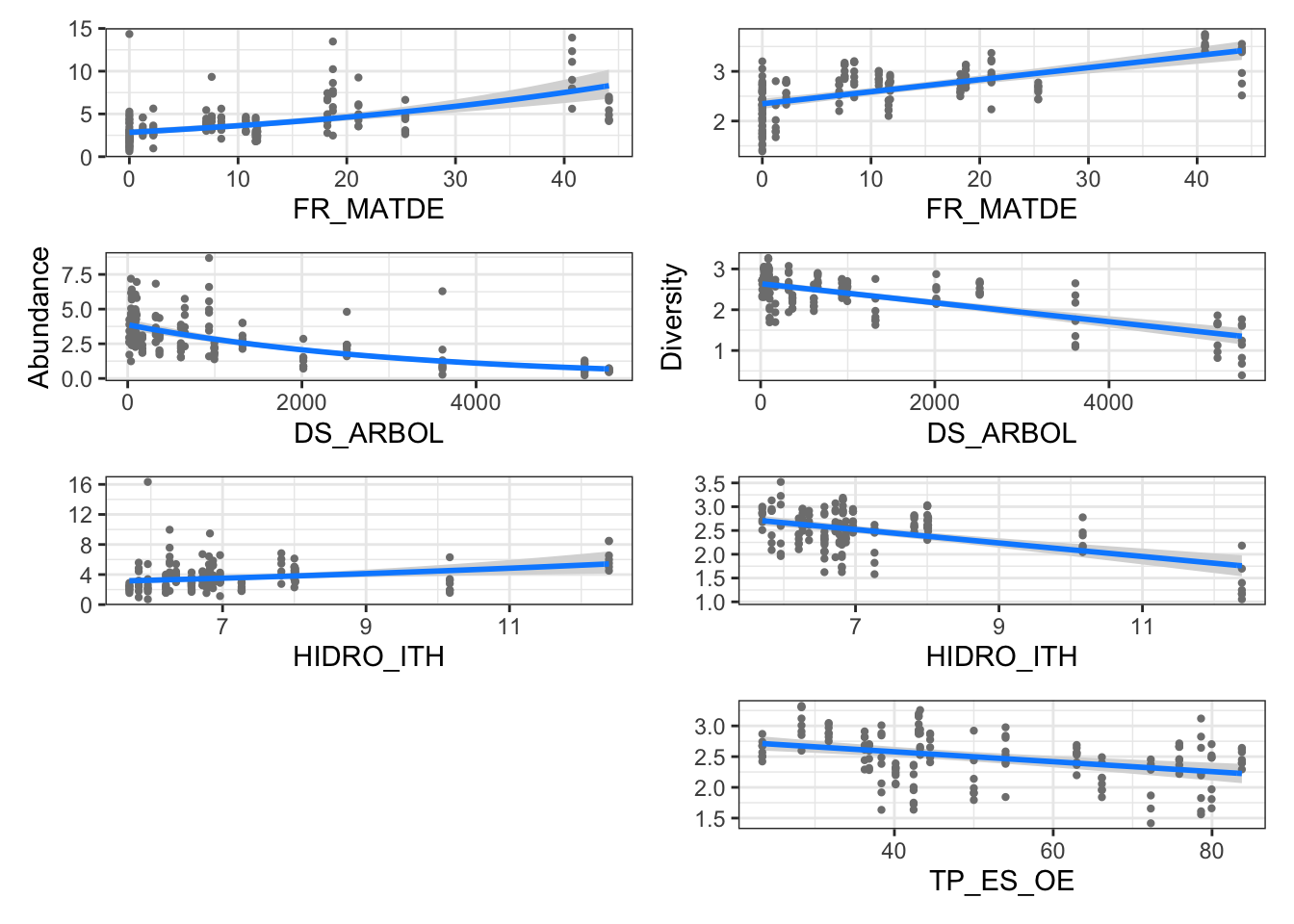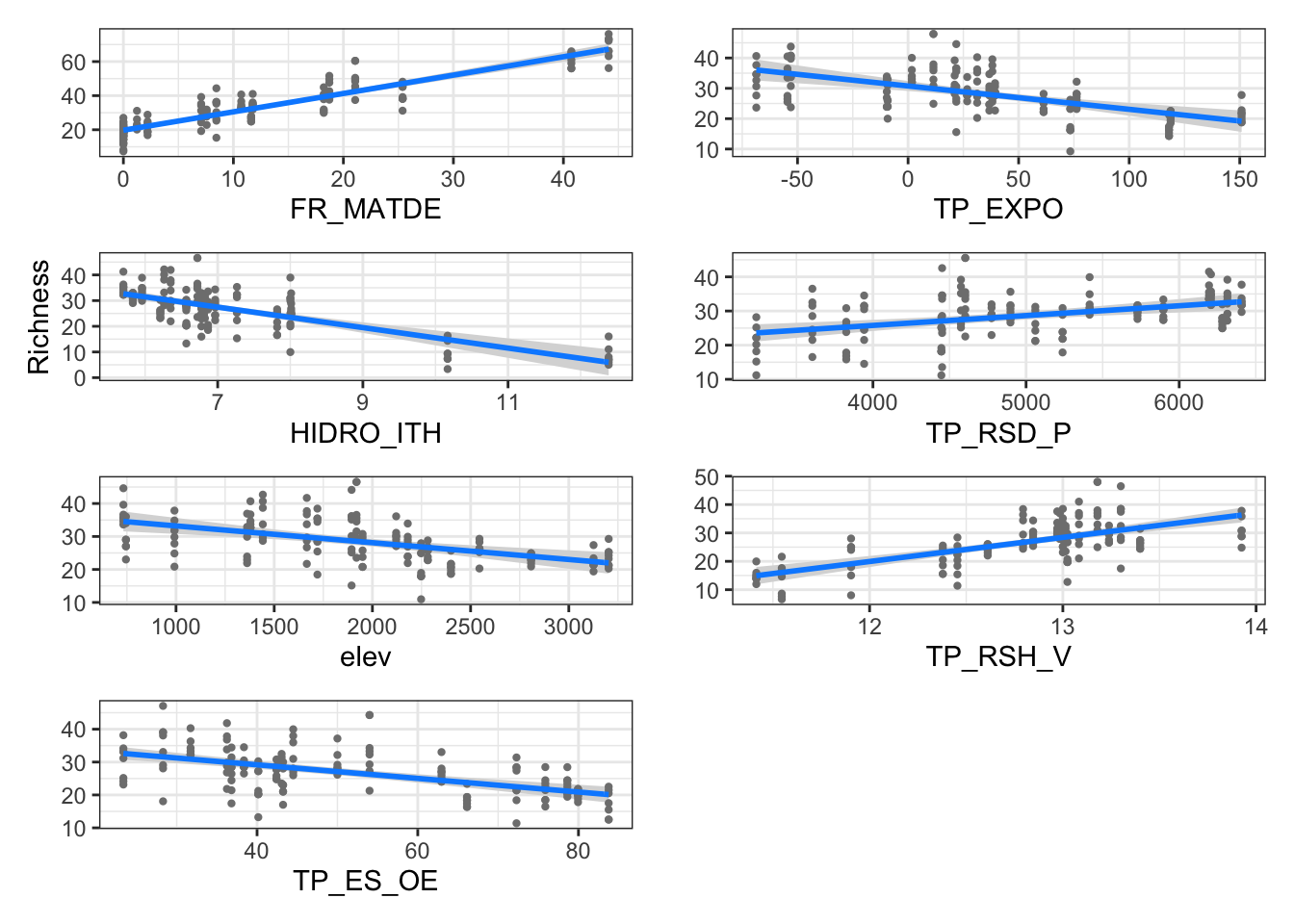Last updated: 2022-01-30

Checks: 7 0

Knit directory: ms_mariposas_biodiversity/

This reproducible R Markdown analysis was created with workflowr (version 1.7.0). The Checks tab describes the reproducibility checks that were applied when the results were created. The Past versions tab lists the development history.

Great! Since the R Markdown file has been committed to the Git repository, you know the exact version of the code that produced these results.

Great job! The global environment was empty. Objects defined in the global environment can affect the analysis in your R Markdown file in unknown ways. For reproduciblity it’s best to always run the code in an empty environment.

The command set.seed(20211228) was run prior to running the code in the R Markdown file. Setting a seed ensures that any results that rely on randomness, e.g. subsampling or permutations, are reproducible.

Great job! Recording the operating system, R version, and package versions is critical for reproducibility.

Nice! There were no cached chunks for this analysis, so you can be confident that you successfully produced the results during this run.

Great job! Using relative paths to the files within your workflowr project makes it easier to run your code on other machines.

Great! You are using Git for version control. Tracking code development and connecting the code version to the results is critical for reproducibility.

The results in this page were generated with repository version e3050d7. See the Past versions tab to see a history of the changes made to the R Markdown and HTML files.

Note that you need to be careful to ensure that all relevant files for the analysis have been committed to Git prior to generating the results (you can use wflow_publish or wflow_git_commit). workflowr only checks the R Markdown file, but you know if there are other scripts or data files that it depends on. Below is the status of the Git repository when the results were generated:


Ignored files:
Ignored:    .Rhistory
Ignored:    .Rproj.user/

Untracked files:
Untracked:  figs/predictors_den_div.pdf
Untracked:  figs/predictors_den_div.png
Untracked:  figs/predictors_rich.pdf

Unstaged changes:
Modified:   analysis/index.Rmd



Note that any generated files, e.g. HTML, png, CSS, etc., are not included in this status report because it is ok for generated content to have uncommitted changes.

These are the previous versions of the repository in which changes were made to the R Markdown (analysis/plots_descriptores.Rmd) and HTML (docs/plots_descriptores.html) files. If you’ve configured a remote Git repository (see ?wflow_git_remote), click on the hyperlinks in the table below to view the files as they were in that past version.

File Version Author Date Message
Rmd e3050d7 ajpelu 2022-01-30 add plots

## Introduction

library(tidyverse)
library(janitor)
library(here)
library(patchwork)
library(vegan)
library(performance)
library(kableExtra)
library(visreg)
library(MASS)
• Prepara datos
diversidad_year <- read_csv(here::here("data/diversidad_by_year.csv"))

dplyr::select(-elev, -temp_anu, -Pp_ver, -Pp_anu)
dplyr::select(Id_transect, year, p_anu_year, p_ver_year, t_anu_year = t_anual)

m <- riqueza_year %>%
dplyr::select(-longitud, -min_altitu, -max_altitu, -long_total, -abundancia) %>%
unite("id", c("id_transecto", "year")) %>%
inner_join(
(climate_year %>% unite("id", c("Id_transect", "year"), remove = FALSE)),
by="id") %>%
inner_join(env) %>%
rowwise() %>%
mutate(FR_ARBOL = sum(FR_CONIF, FR_QUERC)) %>%
relocate(transecto, id, Id_transect, site, elev,year) %>%
dplyr::select(-Transecto, -Abreviatura)

## Modelos

modelo_densidad <- glm(den ~ 1 + FR_MATDE + HIDRO_ITH + DS_ARBOL,
family = Gamma(link ="log"), data = m)

modelo_diversidad <- glm(div ~ 1 + FR_MATDE + HIDRO_ITH + TP_ES_OE + DS_ARBOL, data = m)

modelo_riqueza <- glm(riq ~ 1 + elev + FR_MATDE + TP_RSH_V + HIDRO_ITH + TP_RSD_P + TP_ES_OE + TP_EXPO, data = m)
• Density
d_FR_MATDE <- visreg(modelo_densidad, ylab = "", "FR_MATDE",
scale="response", partial=TRUE, gg=TRUE) + theme_bw()

d_DS_ARBOL <- visreg(modelo_densidad, ylab = "", "DS_ARBOL",
scale="response", partial=TRUE, gg=TRUE) + theme_bw()

d_HIDRO_ITH <- visreg(modelo_densidad, ylab = "", "HIDRO_ITH",
scale="response", partial=TRUE, gg=TRUE) + theme_bw()
• Diveristy
di_FR_MATDE <- visreg(modelo_diversidad, ylab = "", "FR_MATDE",
scale="response", partial=TRUE, gg=TRUE) + theme_bw()

di_DS_ARBOL <- visreg(modelo_diversidad, ylab = "", "DS_ARBOL",
scale="response", partial=TRUE, gg=TRUE) + theme_bw()

di_HIDRO_ITH <- visreg(modelo_diversidad, ylab = "", "HIDRO_ITH",
scale="response", partial=TRUE, gg=TRUE) + theme_bw()

di_TP_ES_OE <- visreg(modelo_diversidad, ylab = "", "TP_ES_OE",
scale="response", partial=TRUE, gg=TRUE) + theme_bw()
• Richness
r_FR_MATDE <- visreg(modelo_riqueza, ylab = "", "FR_MATDE",
scale="response", partial=TRUE, gg=TRUE) + theme_bw()

r_elev <- visreg(modelo_riqueza, ylab = "", "elev",
scale="response", partial=TRUE, gg=TRUE) + theme_bw()

r_TP_RSH_V <- visreg(modelo_riqueza, ylab = "", "TP_RSH_V",
scale="response", partial=TRUE, gg=TRUE) + theme_bw()

r_HIDRO_ITH <- visreg(modelo_riqueza, ylab = "", "HIDRO_ITH",
scale="response", partial=TRUE, gg=TRUE) + theme_bw()

r_TP_RSD_P <- visreg(modelo_riqueza, ylab = "", "TP_RSD_P",
scale="response", partial=TRUE, gg=TRUE) + theme_bw()

r_TP_ES_OE <- visreg(modelo_riqueza, ylab = "", "TP_ES_OE",
scale="response", partial=TRUE, gg=TRUE) + theme_bw()

r_TP_EXPO <- visreg(modelo_riqueza, ylab = "", "TP_EXPO",
scale="response", partial=TRUE, gg=TRUE) + theme_bw()
• All plots
plot_den <- d_FR_MATDE / (d_DS_ARBOL + labs(y="Abundance")) / d_HIDRO_ITH / plot_spacer()

plot_div <- di_FR_MATDE / (di_DS_ARBOL + labs(y="Diversity")) / di_HIDRO_ITH / di_TP_ES_OE

plot_rich <- r_FR_MATDE / r_HIDRO_ITH / r_elev / (r_TP_ES_OE + labs(y="Richness")) / r_TP_EXPO / r_TP_RSD_P / r_TP_RSH_V

den_div <- plot_den | plot_div 
ggsave(here::here("figs/predictors_den_div.pdf"),
device = "pdf",
width = 6, height = 7)
den_divdev.off()
null device
1 
ggsave(plot= den_div,
here::here("figs/predictors_den_div.png"),
device = "png",
dpi = 300,
width = 6, height = 7)
plot_rich_a <- r_FR_MATDE / (r_HIDRO_ITH + labs(y="Richness")) / r_elev / r_TP_ES_OE

plot_rich_b <- r_TP_EXPO / r_TP_RSD_P / r_TP_RSH_V / plot_spacer()

plot_rich_ab <- plot_rich_a | plot_rich_b 
ggsave(here::here("figs/predictors_rich.pdf"),
device = "pdf",
width = 6, height = 7)
plot_rich_abdev.off()
null device
1 
ggsave(plot= plot_rich_ab,
here::here("figs/predictors_den_div.png"),
device = "png",
dpi = 300,
width = 6, height = 7)

sessionInfo()
R version 4.0.2 (2020-06-22)
Platform: x86_64-apple-darwin17.0 (64-bit)
Running under: macOS Catalina 10.15.3

Matrix products: default
BLAS:   /Library/Frameworks/R.framework/Versions/4.0/Resources/lib/libRblas.dylib
LAPACK: /Library/Frameworks/R.framework/Versions/4.0/Resources/lib/libRlapack.dylib

locale:
 en_US.UTF-8/en_US.UTF-8/en_US.UTF-8/C/en_US.UTF-8/en_US.UTF-8

attached base packages:
 stats     graphics  grDevices utils     datasets  methods   base

other attached packages:
 MASS_7.3-53       visreg_2.7.0      kableExtra_1.3.1  performance_0.8.0
 vegan_2.5-7       lattice_0.20-41   permute_0.9-5     patchwork_1.1.1
 tidyr_1.1.3       tibble_3.1.2      ggplot2_3.3.5     tidyverse_1.3.1
 workflowr_1.7.0

loaded via a namespace (and not attached):
 nlme_3.1-152      fs_1.5.0          lubridate_1.7.10  webshot_0.5.2
 insight_0.14.4    httr_1.4.2        rprojroot_2.0.2   tools_4.0.2
 backports_1.2.1   bslib_0.2.4       utf8_1.1.4        R6_2.5.1
 DBI_1.1.1         mgcv_1.8-33       colorspace_2.0-2  withr_2.4.1
 tidyselect_1.1.1  processx_3.5.1    compiler_4.0.2    git2r_0.28.0
 textshaping_0.3.2 cli_2.5.0         rvest_1.0.0       xml2_1.3.2
 labeling_0.4.2    sass_0.3.1        scales_1.1.1.9000 callr_3.7.0
 systemfonts_1.0.0 digest_0.6.27     rmarkdown_2.8     pkgconfig_2.0.3
 htmltools_0.5.2   highr_0.8         dbplyr_2.1.1      fastmap_1.1.0
 rlang_0.4.12      rstudioapi_0.13   farver_2.1.0      jquerylib_0.1.3
 generics_0.1.0    jsonlite_1.7.2    magrittr_2.0.1    Matrix_1.3-2
 Rcpp_1.0.7        munsell_0.5.0     fansi_0.4.2       lifecycle_1.0.1
 stringi_1.7.4     whisker_0.4       yaml_2.2.1        snakecase_0.11.0
 grid_4.0.2        parallel_4.0.2    promises_1.2.0.1  crayon_1.4.1
 haven_2.3.1       splines_4.0.2     hms_1.0.0         knitr_1.31
 ps_1.5.0          pillar_1.6.1      reprex_2.0.0      glue_1.4.2
 evaluate_0.14     getPass_0.2-2     modelr_0.1.8      vctrs_0.3.8
 httpuv_1.5.5      cellranger_1.1.0  gtable_0.3.0      assertthat_0.2.1
 xfun_0.23         broom_0.7.9       later_1.1.0.1     ragg_1.1.1
 viridisLite_0.4.0 cluster_2.1.0     ellipsis_0.3.2